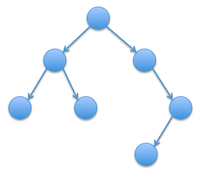## Part 1: Measuring Inefficiency (30%)

In class, we analyzed the `add` method for the BinaryTree class. Because each recursive call involves counting the number of nodes in each subtree (an O(N) operation) and the number of calls depends upon the height of the tree (which will be roughly log N in a balanced tree), adding an item using this method will be O(N log N). Design a program that will verify this analysis experimentally using techniques similar to HW3. Provide statistics from executions of your program and a coherent argument as to why the statistics support the claim that `add` is O(N log N).

## Part 2: Inductive Proofs (30%)

The height of a tree is defined to be the length of the longest path (in terms of number of nodes) from the root to a leaf. The weight of a tree is defined to be the sum of all the node depths. For example, the height of the tree shown below is 4 while its weight is 1 + 2 + 2 + 3 + 3 + 3 + 4 = 18.Both of these properties can also be defined using recurrence relations. An empty tree is assumed to have 0 as its height and weight. Otherwise, for nonempty binary tree `T`:

height(T) = max(height(left subtree of T), height(right subtree of T)) + 1 weight(T) = weight(left subtree of T) + weight(right subtree of T) + size(T)

where `size(T)` is the number of nodes in tree `T`. For each of these recurrence relations, provide an inductive proof that the relation does actually hold.

## Part 3: Binary Tree Methods (40%)

Add methods to the BinaryTree class named `height` and `weight`, that calculate and return these tree properties. Initially, you may use the provided `size` method to test your code. Once it is working, replace the `size` method with a more efficient version that makes use of a field to keep track of the number of nodes in the tree. That is, the field should be initialized to 0 and incremented/decremented each time an add/remove is executed.

Modify the main method of your `BinaryTree` class so that it tests each of these methods. Part of your grade will depend upon the thoroughness of your tests.